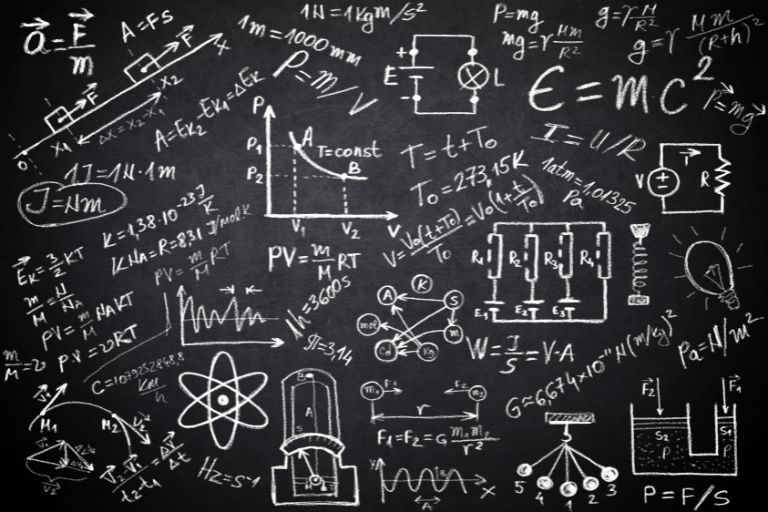# How do we understand concepts in Physics?

September 8, 2022Many students struggle with grasping concepts in Physics, especially if they struggle with other related subjects such as Mathematics. Here are some tips on how to improve a student’s understanding of Physics at the secondary school and Junior College level!

### Visualisation of Physics Concepts

Visualising the concepts in real life is incredibly important. When dealing with topics such as the forces acting on an object or the refraction of light, it is difficult to imagine how objects move when a student is merely staring at the question on a paper. A hands-on demonstration can be helpful to the student. A student does not need to obtain the materials necessary to perform a live demonstration as there are online tools and models which can portray the topic well.

Often, students only have a theoretical understanding of Physics, which limits their ability to answer questions, especially questions with a new pattern or a new set of information which tests the student’s knowledge in a way which has not been seen in past questions. By visualising the concepts, a student will be able to use his or her common sense to figure out how the forces act upon an object, for example. It is highly recommended for students to try and view the real-life application of these physical concepts so that they truly understand them, instead of memorising all the permutations of the sample questions.

### Application of Physics Formulas

The study of Physics involves many formulas which students need to remember. Students having trouble remembering the formulas should understand how the elements in the formulas are related to one another.

Although it is good to understand how to derive certain formulas, students should focus more on understanding what these equations are trying to say in a fundamental sense. It is not enough to simply manipulate these equations according to the example to obtain the correct answer. Rather, students should try to understand the context in which these equations are formed.

If we take the simplest example of the equation for speed, which is the distance over time, students who memorise the equation as Speed = distance/time sometimes fail to understand what the concept of speed is. What is speed? How fast can I get somewhere? What does the getting somewhere involve? Getting somewhere involves traveling. Is it good enough to just state the time it takes to get somewhere? It is not enough, as there is the question of where it is I am trying to go. Therefore, speed is how fast I can travel a certain journey, which gives me the distance over time.

Breaking down formulas into simpler explanations using logic and common sense could help students visualise and understand concepts in Physics.

### Understanding Sample Questions

An important aspect of scoring well in Physics is to understand the demands of the question. A useful approach students could take is to refer to the model answers and work backwards, figuring out why the model answer says something and thinking about how to tailor their answers to suit the model answer. This is a good approach for students who are already closer to their exams, who may not have the time to undertake learning from the ground-up, as opposed to students who have just started to learn Physics. This approach could suit certain types of papers, such as practical papers which deal with experiments, as there are fewer permutations of questions to study for, as opposed to the more content-heavy papers.

To conclude, an improvement in Physics requires not only hard work from students but an ability to identify what the student does not know about the subject and how to better arrive at the answer.